Goalist Developers Blog

# Scan documents using OpenCV python

こんにちは、 ゴーリストのビベックです。 Hello World! This is Vivek from Goalist.

In this blog post, let's play around OpenCV library and write our own python script to scan documents like receipts, business cards, pages of book etc.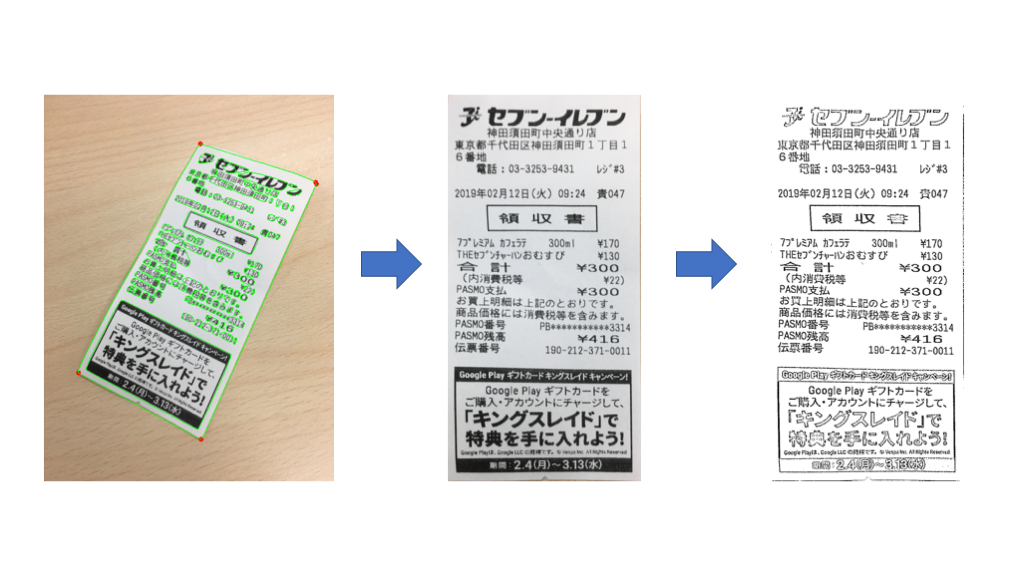What is OpenCV?
OpenCV (Open Source Computer Vision) is a library of programming functions mainly aimed at real-time computer vision. The library is cross-platform and free for use under the open-source BSD license. OpenCV supports the deep learning frameworks TensorFlow, PyTorch, and Caffe.

What OpenCV can do?
2. Detection of faces and its features
3. Detection of shapes like Circle, rectangle etc in an image
4. Text recognition in images
5. Modifying image quality and colors
6. Developing Augmented reality apps
and much more.....

Which Languages does OpenCV support?
1. C++
2. Python
3. Java
4. Matlab/Octave
5. C
6. There are wrappers in other languages like Javascript, C#, Perl, Haskell, and Ruby to encourage adoption by a wider audience.

The initial version of OpenCV was released in June 2000, that does mean; (at the time of writing this post) it's almost 19 years this library is in use.

Some papers also highlight the fact that OpenCV was built to provide a common infrastructure for computer vision applications and to accelerate the use of machine perception in commercial products.

So let's get started and let's see what we can build with it...

### Step 1: Setting up the environement

We will be using Python 3 for our project, so, ensure that you have Python version 3 as your development environment.
You may refer the following link to set up Python on your machine.

### Step 2: Gather required packages

We will be needing following packages in our project
1) Pre-built OpenCV packages for Python
`opencv-python==4.0.0.21`
`opencv-contrib-python==4.0.0.21`

2) For Array computation
`numpy==1.16.1`

3) For applying filters to image (image processing)
`scikit-image==0.14.2`

4) Utility package for image manupulation
`imutils==0.5.2`

### Step 3: Let's make it work

Import the installed packages into your python script

```import cv2 # opencv-python
import numpy as np
from skimage.filters import threshold_local # scikit-image
import imutils
```

Read the image to be scanned into your script by using OpenCV's `imread()` function.

We are going to perform edge detection on the input image hence in order to increase accuracy in edge detection phase we may want to resize the image. So, compute the `ratio` of the old height to the new height and `resize()` it using `imutils`

Also keep the cloned copy of `original_image` for later use

```# read the input image

# clone the original image
original_image = image.copy()

# resize using ratio (old height to the new height)
ratio = image.shape / 500.0
image = imutils.resize(image, height=500)
```

Generally paper (edges, at least) is white so you may have better luck by going to a different color space like YUV which better separates luminosity. (Read more about this here YUV - Wikipedia )
In order to change the color space of the input image use OpenCV's `cvtColor()` function.
From YUV image let's get rid of chrominance {color} (UV) components and only use luma {black-and-white} (Y) component for further proccesing.

```#  change the color space to YUV
image_yuv = cv2.cvtColor(image, cv2.COLOR_BGR2YUV)

# grap only the Y component
image_y = np.zeros(image_yuv.shape[0:2], np.uint8)
image_y[:, :] = image_yuv[:, :, 0]
```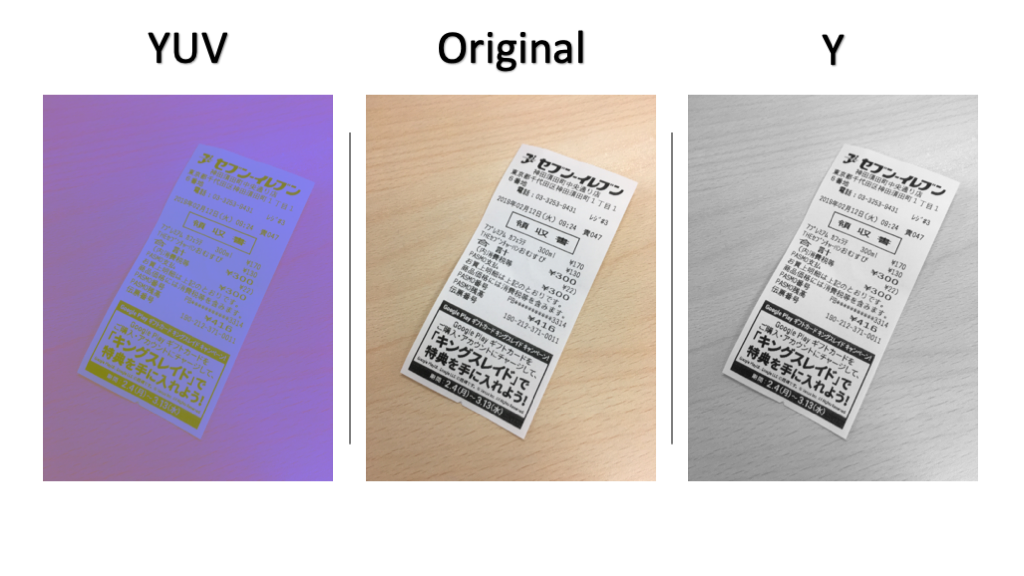The text on the paper is another problem while detecting edges so let's use blurring effect `GaussianBlur()`, to remove these high-frequency noises (hopefully to some extent)

```# blur the image to reduce high frequency noises
image_blurred = cv2.GaussianBlur(image_y, (3, 3), 0)
```

It's time to detect edges in our input image.
Use `Canny()` function to detect edges. You may have to tweak threshold parameters of this function in order to get the desired output.

```# find edges in the image
edges = cv2.Canny(image_blurred, 50, 200, apertureSize=3)
```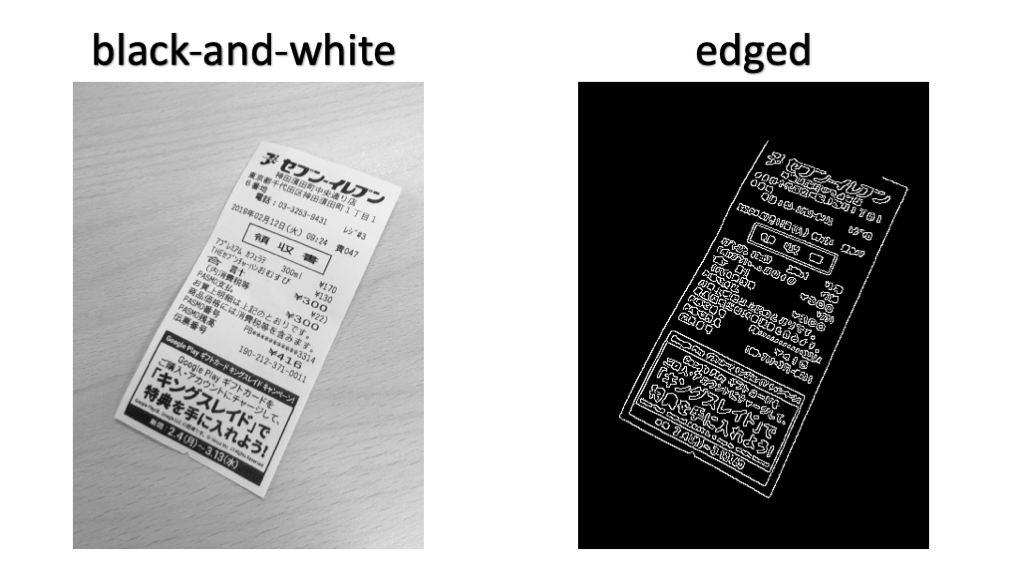Now that we have detected edges in our input image let's find contours around the edges and draw it on the original image

```# find contours
contours, hierarchy = cv2.findContours(edges, cv2.RETR_EXTERNAL, cv2.CHAIN_APPROX_SIMPLE)

# draw all contours on the original image
cv2.drawContours(image, contours, -1, (0, 255, 0), 1)
# !! Attention !! Do not draw contours on the image at this point
# I have drawn all the contours just to show below image
```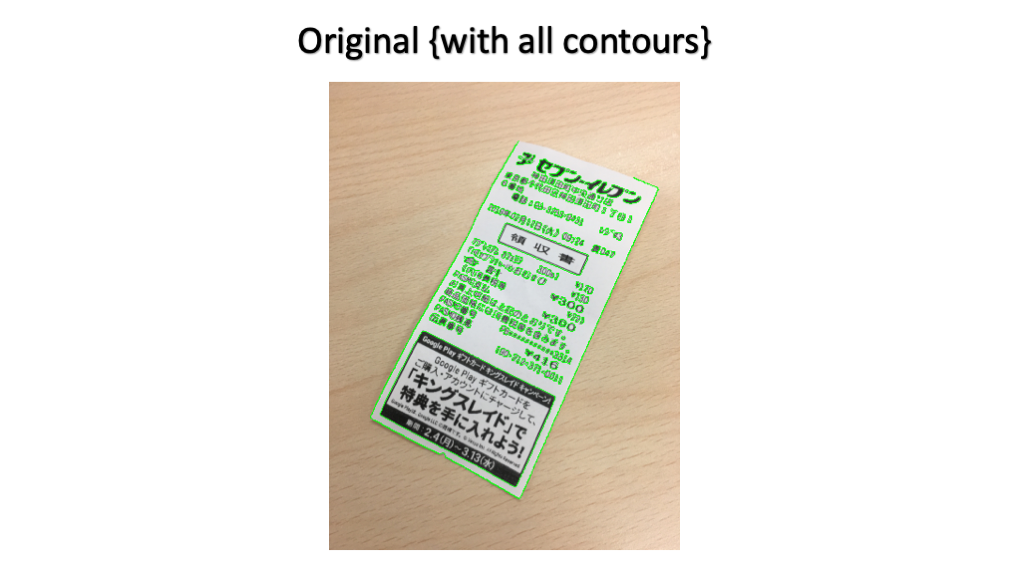Now that we should have a bunch of contours with us, it's time to find the right ones.
For each contour `cnt`, first, find the Convex Hull (Convex hull - Wikipedia), then use `approaxPolyDP` to simplify the contour as much as possible.

```# to collect all the detected polygons
polygons = []

# loop over the contours
for cnt in contours:
# find the convex hull
hull = cv2.convexHull(cnt)

# compute the approx polygon and put it into polygons
polygons.append(cv2.approxPolyDP(hull, 0.01 * cv2.arcLength(hull, True), False))
```

Sort the detected polygons in the descending order of contour area so that we will get a polygon with the largest areas found inside the image

```# sort polygons in desc order of contour area
sortedPoly = sorted(polygons, key=cv2.contourArea, reverse=True)

# draw points of the intersection of only the largest polyogon with red color
cv2.drawContours(image, sortedPoly, -1, (0, 0, 255), 5)
```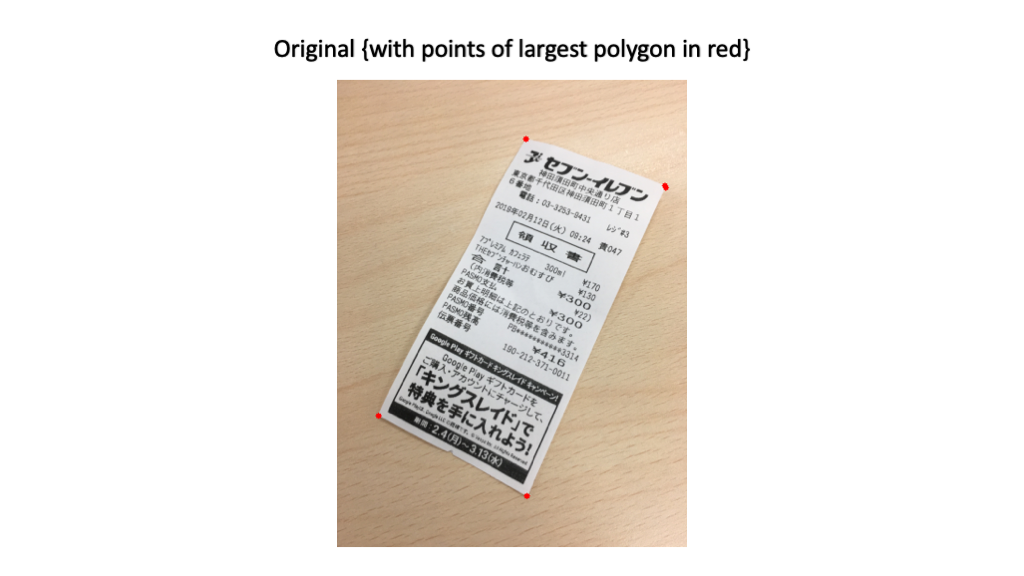We now check if the largest detected polygon has four points.
If the polygon has four points congratulations we have detected four corners of the document in the image.

It's time to crop the image and transform the perspective of the image with respect to these four points

```# get the contours of the largest polygon in the image
simplified_cnt = sortedPoly

# check if the polygon has four point
if len(simplified_cnt) == 4:
# trasform the prospective of original image
cropped_image = four_point_transform(original_image, simplified_cnt.reshape(4, 2) * ratio)
```

Refer the following to get to know about `four_point_transform()` function in detail.

Finally binarize the image to have scanned version of the cropped image

```# Binarize the cropped image
gray_image = cv2.cvtColor(cropped_image, cv2.COLOR_BGR2GRAY)
T = threshold_local(gray_image, 11, offset=10, method="gaussian")
binarized_image = (gray_image > T).astype("uint8") * 255

# Show images
cv2.imshow("Original", original_image)
cv2.imshow("Scanned", binarized_image)
cv2.imshow("Cropped", cropped_image)
cv2.waitKey(0)
```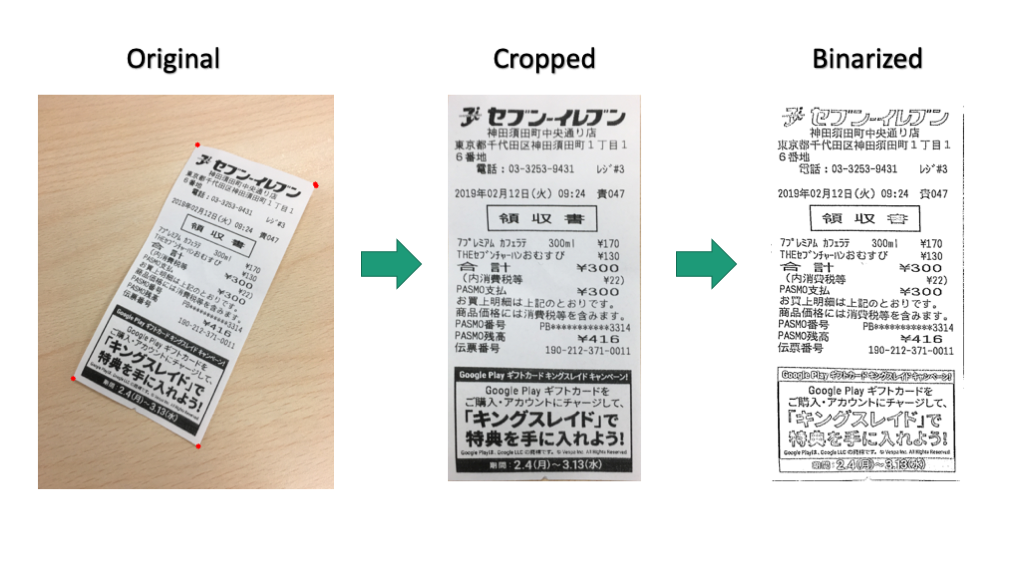🎉There we go... we just managed to scan a document from a raw image with the help of OpenCV.

That's all for this post see you soon with one of such next time; until then,
Happy Learning :)